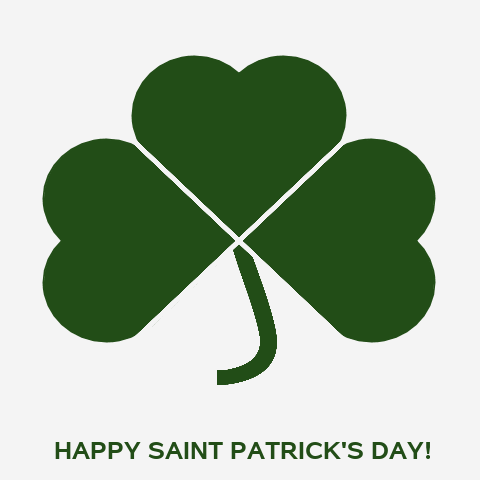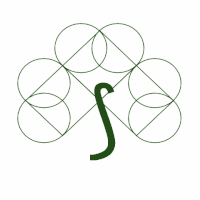## Fun with SAS ODS Graphics: A Heart-felt Happy St. Patrick's Day to All!Recycled last month's Square + Circles Valentine code to wish everyone Happy St. Patrick's Day!BeforeAfter

``````* Fun with SAS ODS Graphics: A Heart-felt Happy St. Patrick's Day to All!

Animated GIF of a "shamrock" made up of three heart-shaped leaves (each consisting of two
circles and a square rotated 45 degrees) and a math symbol "stem" (a "right s-shaped bag");

data points;                                       * Generate points for chart;
stem=unicode('\u27c6'); xT=.075; yT=1.5*-sin(constant("pi")/4); * Unicode math symbol ("right s-shaped bag") used for shamrock stem;
xO=0; yO=-sin(constant("pi")/4);                   * Points for 45-degree lines that will be used to divide the 3 "hearts" of the shamrock;
* Generate points for left heart-shaped "leaf" (rotated counterclockwise 90 degrees);
square=1;                                          * 1 square, sides of length 1, rotated 45 degrees;
xs=0; ys=-sin(constant("pi")/4); output;
xs=-cos(constant("pi")/4); ys=2*-sin(constant("pi")/4); output;
xs=2*-cos(constant("pi")/4); ys=-sin(constant("pi")/4); output;
xs=-cos(constant("pi")/4); ys=0; output;
xs=.; square=.;                                     * Reset square variables to missing;
circle=1;                                           * 2 circles of diameter 1, centered on top left/ride sides of square;
x=-1.5*cos(constant("pi")/4); y=-3*sin(constant("pi")/4)/2; output;
circle=2; y=-sin(constant("pi")/4)/2; output;
x=.; y=.; circle=.;                                 * Reset circle variables to missing;

square=2;                                           * Essentially repeat above for center heart-shaped "leaf" (no rotation);
xs=-cos(constant("pi")/4); ys=0; output;
xs=0; ys=sin(constant("pi")/4); output;
xs=cos(constant("pi")/4); ys=0; output;
xs=0; ys=-sin(constant("pi")/4); output;
xs=.; square=.;
circle=3; x=-cos(constant("pi")/4)/2; y=sin(constant("pi")/4)/2; output;
circle=4; x=-x; output;
x=.; y=.; circle=.;

square=3;                                            * Essentially repeat above for right heart-shaped "leaf" (rotated clockwise 90 degrees);
xs=0; ys=-sin(constant("pi")/4); output;
xs=cos(constant("pi")/4); ys=2*-sin(constant("pi")/4); output;
xs=2*cos(constant("pi")/4); ys=-sin(constant("pi")/4); output;
xs=cos(constant("pi")/4); ys=0; output;
xs=.; square=.;
circle=5; x=1.5*cos(constant("pi")/4); y=-sin(constant("pi")/4)/2; output;
circle=6; y=-3*sin(constant("pi")/4)/2; output;
x=.; y=.; circle=.;

data squares; set points(where=(square^=.));         * Grab points for squares;
data circles; set points(where=(circle^=.));         * Grab points for circles;
data shapes; set squares circles;                    * Merge back together in desired potting order - squares first, then cirlcles;

%macro genframes;                                    /* Generate 22 frame Happy St. Patrick's Day! animated GIF */
%do i=0 %to 22;
%if &i=0 %then %let f=22; %else %let f=&i;           /* Show complete greeting at beginning and end of looping GIF */
data frame;                                          /* Select data for frame */
xsf=.; ysf=.; xf=.; yf=.; circlef=.;                 /* *f macro vars used for coordinates of filled shapes */
set shapes;
%if 1<=&f<=10 %then                                  /* In frames 1-10, introduce unfilled 3 squares, unfilled 6 circles, and math symbol one-by-one */
where (square^=. & square<=&f) or (circle^=. & circle<=&f-3);;
%if &f>=11 %then %do;                                /* Squares and circles plotted first without fill, then with fill */
if square^=. and &f>=10+square then                  /* Fill circles and squares one-by-one */
do; xsf=xs; ysf=ys; xs=.; ys=.; end;               /* Put values in variables used by filled squares polygon plot */
if circle^=. and &f>=13+circle then
do; xf=x; yf=y; x=.y=.; circlef=circle; circle=.; end;  /* Put values in variables used by filled circles ellipse plot */
%end;

proc sgplot data=frame aspect=1 noautolegend nowall noborder; /* Draw the shamrock animation frames */
%if &f>=20 %then styleattrs backcolor=cxF5F5F5;;              /* Fill background with very light green near end of animation */
polygon x=xs y=ys id=square / outline lineattrs=(color=cx224d17 thickness=1.75pt) nofill; /* Unfilled squares */
polygon x=xsf y=ysf id=square / outline lineattrs=(color=cx224d17 thickness=1.75pt) fill fillattrs=(color=cx224d17); /* Filled sqquares */
ellipseparm semimajor=.5 semiminor=.5 / xorigin=x yorigin=y group=circle nomissinggroup
outline lineattrs=(color=cx224d17 thickness=1.75pt) nofill; /* Unfilled circles */
ellipseparm semimajor=.5 semiminor=.5 / xorigin=xf yorigin=yf group=circlef nomissinggroup
outline lineattrs=(color=cx224d17 thickness=1.75pt) fill fillattrs=(color=cx224d17); /* Filled circles */
%if &f>=10 %then                                               /* Use Unicode math symbol for shamrock "stem" */
text x=xT y=yT text=stem / textattrs=(size=144pt color=cx224d17 family="unicode arial ms") contributeoffsets=none;;
%if &f>=21 %then %do;                                          /* Draw lines to better highlight the 3 'heart-shaped' leaves */
lineparm x=xO y=yO slope=1 / lineattrs=(color=cxF5F5F5 thickness=3pt);
lineparm x=xO y=yO slope=-1 / lineattrs=(color=cxF5F5F5 thickness=3pt);
%end;
xaxis display=none values=(-1.8 1.8);                          /* Scale axes and suppress their display */
yaxis display=none values=(-2.6 1.2);
%if &f=22 %then                                                /* Display Happy St. Patrick's Day on last frame */
inset "HAPPY SAINT PATRICK'S DAY!" / position=bottom textattrs=(color=cx224d17 weight=bold size=18pt);;
run;
options animduration=.33;                                      /* Display first frame for 3.7 seconds, others for 1/3 second */
%end;
%mend;
* Create animated GIF from frames;
options papersize=('5 in', '5 in') printerpath=gif animation=start
nodate nonumber animloop=YES animduration=3.5 NOANIMOVERLAY;
ods printer file='~/HappyStPatricksDay2022.gif';
ods graphics / reset antialias border=no height=5in width=5in imagefmt=gif border=off;
%genframes;                                                    * Generate the 22 frames of images;
options printerpath=gif animation=stop;                        * Wrap-up animated GIF creation;
run;
ods printer close;``````
Discussion stats
• 0 replies
• 477 views
• 5 likes
• 1 in conversation